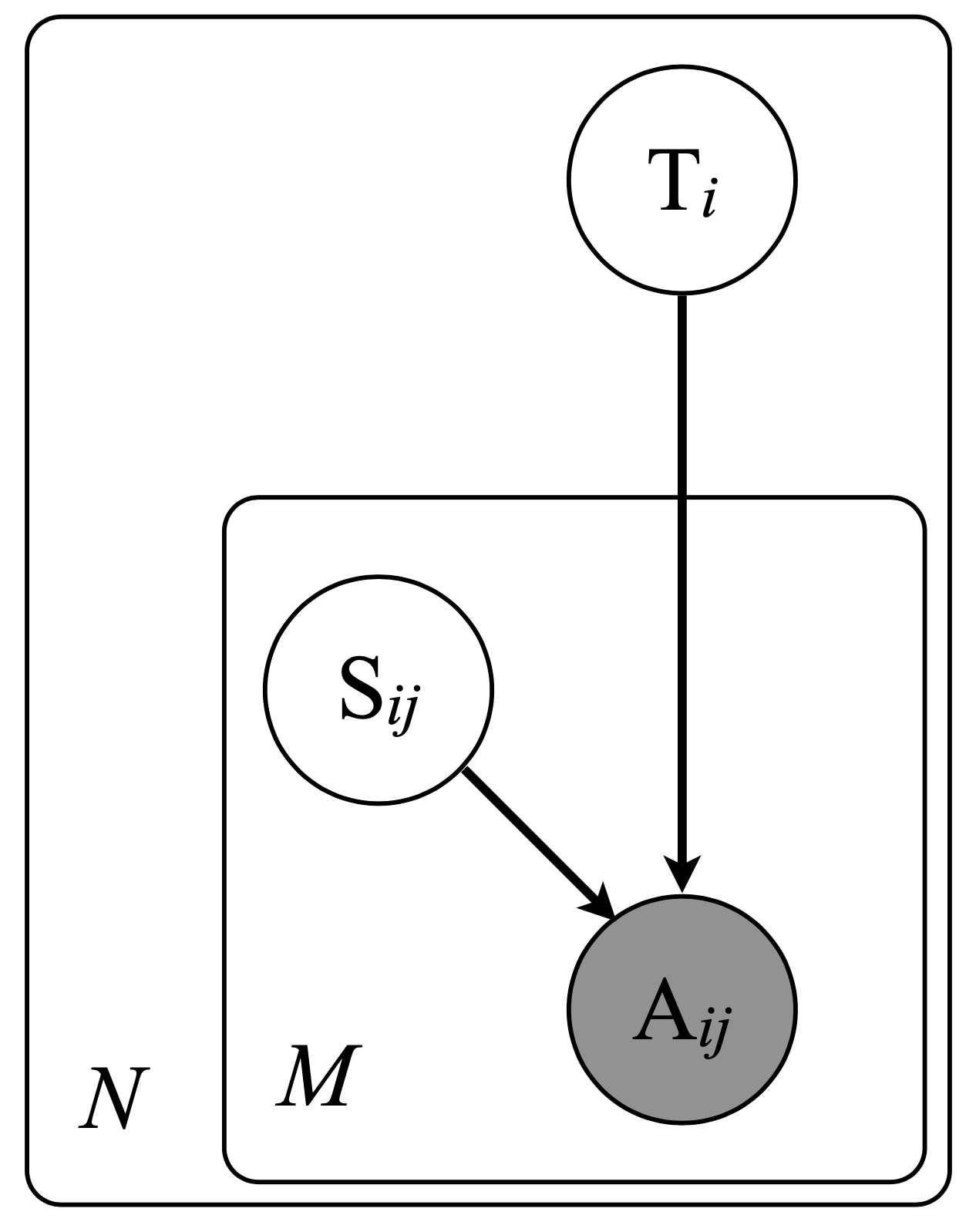# MACE

crowdkit.aggregation.classification.mace.MACE | Source code

MACE(
self,
n_restarts: int = 10,
n_iter: int = 50,
method: str = 'vb',
default_noise: float = 0.5,
alpha: float = 0.5,
beta: float = 0.5,
random_state: int = 0,
verbose: int = 0
)


Multi-Annotator Competence Estimation.

Probabilistic model that associates each worker with a probability distribution over the labels. For each task, a worker might be in a spamming or not spamming state. If the worker is not spamming, they yield a correct label. If the worker is spamming, they answer according to their probability distribution.

We assume that the correct label $T_i$ comes from a discrete uniform distribution. When a worker annotates the task, they are in the spamming state with probability $\operatorname{Bernoulli}(1 - \theta_w)$. So, if their state $s_w = 0$, their response $A_{iw} = T_i$. Otherwise, their response $A_{iw}$ is drawn from a multinomial distribution with parameters $\xi_w$.The model can be enhanced by adding a Beta prior over $\theta_w$ and Diriclet prior over $\xi_w$.

D. Hovy, T. Berg-Kirkpatrick, A. Vaswani and E. Hovy. Learning Whom to Trust with MACE. In Proceedings of NAACL-HLT, Atlanta, GA, USA (2013), 1120–1130.

https://aclanthology.org/N13-1132.pdf

## Parameters Description

Parameters Type Description
n_restarts int

The of algorithms optimization runs. The final parameters are ones that gave the best log likelihood. When a single run takes too long, it is fine to set this parameter to 1. Default: 10.

n_iter int

The number of EM iterations for each optimization run. Default: 50.

method str

The method to use for the M-step. Either 'vb' or 'em'. 'vb' means optimization through variational Bayes using priors. 'em' stands for straightforward Expectation-Maximization. Default: 'vb'.

smoothing -

The smoothing parameter for the normalization. Default: 0.1.

alpha float

The prior parameter for the Beta distribution over $\theta_w$. Default: 0.5.

beta float

The prior parameter for the Beta distribution over $\theta_w$. Default: 0.5.

default_noise float

The default noise parameter for the initialization. Default: 0.5.

verbose int

Whether to print progress. 0 — no progress bar, 1 — only for restarts, 2 — for both restarts and optimization. Default: 0.

labels_ Optional[Series]

Tasks' labels. A pandas.Series indexed by task such that labels.loc[task] is the tasks's most likely true label.

probas_ Optional[DataFrame]

Tasks' label probability distributions. A pandas.DataFrame indexed by task such that result.loc[task, label] is the probability of task's true label to be equal to label. Each probability is between 0 and 1, all task's probabilities should sum up to 1

spamming_ ndarray

Posterior distribution of workers' spamming states.

thetas_ ndarray

Posterior distribution of workers' spamming labels.

Examples:

from crowdkit.aggregation import MACE